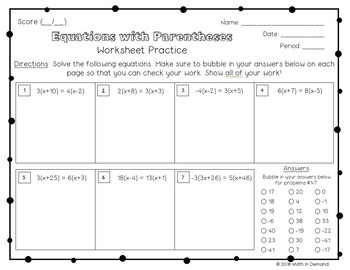# 8th Grade Math Practice Worksheets (Entire Year of Worksheets)

Rated 5 out of 5, based on 12 reviews
12 Ratings;
8th
Subjects
Resource Type
Formats Included
• Zip
Pages
\$39.99
\$39.99
Report this resource to TPT
##### Also included in
1. ⭐ This bundle includes ALL of my 8th Grade Math material in my store and ALL future 8th Grade Math Products ⭐This includes all of my popular 8th grade math interactive notebooks, practice worksheets, foldables, activities, warm-ups, homework, exit slips, assessments, early finishers, and SO MUCH MOR
Price \$249.99Original Price \$495.37Save \$245.38
2. ⭐ This bundle includes ALL of my Math Curriculum in my Teachers Pay Teachers store ⭐You will be receiving ALL of my 6th, 7th, and 8th Grade Math interactive notebooks, activities, foldables, practice worksheets, warm-ups, exit slips, assessments, and much more! This is well over 1000 pages of materi
Price \$899.99Original Price \$1718.65Save \$818.66
3. This listing is for a whole years worth of practice worksheets for 6th, 7th, and 8th grade math (some worksheets are still in progress). That is 163 math worksheets when all worksheets are completed!!! These worksheets are self assessing because they include a bubble-in area for the answer key. Som
Price \$74.99Original Price \$119.97Save \$44.98

### Description

There is a whole years worth of practice worksheets for 8th grade math. There are 55 worksheets that are self-assessing because they include a bubble-in area for the answer key. I have broken up my worksheets into 5 units:

The units include:

Unit 1 - The Number System

Unit 2 - Expressions & Equations

Unit 3 - Functions

Unit 4 - Geometry

Unit 5 - Statistics & Probability

A breakdown of each unit is below. There is one worksheet for each concept below:

Unit 1 - The Number System

-The Real Number System

-Classifying: Irrationals vs Rationals

-Converting a Fraction into a Repeating Decimal

-Converting a Repeating Decimal into a Fraction

-Square Roots and Cube Roots

-Approximating Non-Perfect Squares

-Non-Perfect Squares on the Number Line

-Comparing Irrational Numbers

-Exponent Properties

-Intro to Scientific Notation

-Multiplying/Dividing with Scientific Notation

Unit 2 - Expressions & Equations

-Unit Rate on a Graph

-Solving Equations with Variables on Both Sides

-Equations with Parentheses

-Number of Solutions to Linear Equations

-X and Y intercepts from a Table and Graph

-X and Y intercepts from an Equation

-Graphing using Intercepts

-Types of Slope

-Determining Slope Given a Graph

-Determining Slope Given a Table or Two Points

-Slope-Intercept Form

-Writing Linear Equations from a Graph

-Writing Linear Equations Given Two Points

-Standard Form to Slope-Intercept Form

-Types of Solutions from a Systems of Equations

-Solving Systems of Equations by Graphing

-Solving Systems of Equations by Substitution

-Solving Systems of Equations by Elimination

-Solving Systems of Equations Word Problems

Unit 3 - Functions

-Intro to Functions

-Function Notation and Evaluating a Function

-Linear vs Non-Linear

-Writing Linear Functions from a Table

-Comparing Functions (Graph, Table, Equation, and Verbal Description)

Unit 4 - Geometry

-Intro to Transformations

-Rigid Transformations: Reflections, Rotations, & Translations Rules

-Dilations

-Sequence of Transformations

-Similarity

-Congruence

-Identifying Angle Relationships

-Solving with Angle Relationships

-Triangle Angle Sum Theorem

-Triangle Exterior Angle Theorem

-Proving the Pythagorean Theorem

-Applying the Pythagorean Theorem

-Pythagorean Theorem in Coordinate System

-Volume: Cone, Cylinder, and Sphere

Unit 5 - Statistics & Probability

-Intro to Scatter Plots

-Constructing Scatter Plots

-Line of Best Fit

-Two Way Tables

If you like my worksheets, then you should check out my 8th Grade Math Interactive Notebook. The notebook and worksheets are aligned perfectly.

Total Pages